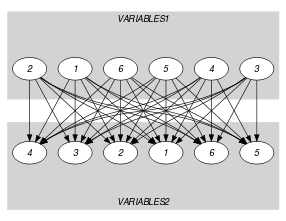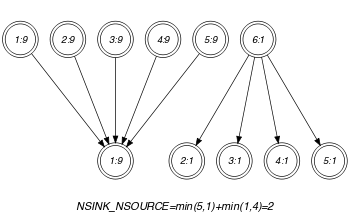## 5.362. soft_same_interval_var

Origin
Constraint

$\mathrm{𝚜𝚘𝚏𝚝}_\mathrm{𝚜𝚊𝚖𝚎}_\mathrm{𝚒𝚗𝚝𝚎𝚛𝚟𝚊𝚕}_\mathrm{𝚟𝚊𝚛}\left(𝙲,\mathrm{𝚅𝙰𝚁𝙸𝙰𝙱𝙻𝙴𝚂}\mathtt{1},\mathrm{𝚅𝙰𝚁𝙸𝙰𝙱𝙻𝙴𝚂}\mathtt{2},\mathrm{𝚂𝙸𝚉𝙴}_\mathrm{𝙸𝙽𝚃𝙴𝚁𝚅𝙰𝙻}\right)$

Synonym

$\mathrm{𝚜𝚘𝚏𝚝}_\mathrm{𝚜𝚊𝚖𝚎}_\mathrm{𝚒𝚗𝚝𝚎𝚛𝚟𝚊𝚕}$.

Arguments
 $𝙲$ $\mathrm{𝚍𝚟𝚊𝚛}$ $\mathrm{𝚅𝙰𝚁𝙸𝙰𝙱𝙻𝙴𝚂}\mathtt{1}$ $\mathrm{𝚌𝚘𝚕𝚕𝚎𝚌𝚝𝚒𝚘𝚗}\left(\mathrm{𝚟𝚊𝚛}-\mathrm{𝚍𝚟𝚊𝚛}\right)$ $\mathrm{𝚅𝙰𝚁𝙸𝙰𝙱𝙻𝙴𝚂}\mathtt{2}$ $\mathrm{𝚌𝚘𝚕𝚕𝚎𝚌𝚝𝚒𝚘𝚗}\left(\mathrm{𝚟𝚊𝚛}-\mathrm{𝚍𝚟𝚊𝚛}\right)$ $\mathrm{𝚂𝙸𝚉𝙴}_\mathrm{𝙸𝙽𝚃𝙴𝚁𝚅𝙰𝙻}$ $\mathrm{𝚒𝚗𝚝}$
Restrictions
 $𝙲\ge 0$ $𝙲\le |\mathrm{𝚅𝙰𝚁𝙸𝙰𝙱𝙻𝙴𝚂}\mathtt{1}|$ $|\mathrm{𝚅𝙰𝚁𝙸𝙰𝙱𝙻𝙴𝚂}\mathtt{1}|=|\mathrm{𝚅𝙰𝚁𝙸𝙰𝙱𝙻𝙴𝚂}\mathtt{2}|$ $\mathrm{𝚛𝚎𝚚𝚞𝚒𝚛𝚎𝚍}$$\left(\mathrm{𝚅𝙰𝚁𝙸𝙰𝙱𝙻𝙴𝚂}\mathtt{1},\mathrm{𝚟𝚊𝚛}\right)$ $\mathrm{𝚛𝚎𝚚𝚞𝚒𝚛𝚎𝚍}$$\left(\mathrm{𝚅𝙰𝚁𝙸𝙰𝙱𝙻𝙴𝚂}\mathtt{2},\mathrm{𝚟𝚊𝚛}\right)$ $\mathrm{𝚂𝙸𝚉𝙴}_\mathrm{𝙸𝙽𝚃𝙴𝚁𝚅𝙰𝙻}>0$
Purpose

Let ${N}_{i}$ (respectively ${M}_{i}$) denote the number of variables of the collection $\mathrm{𝚅𝙰𝚁𝙸𝙰𝙱𝙻𝙴𝚂}\mathtt{1}$ (respectively $\mathrm{𝚅𝙰𝚁𝙸𝙰𝙱𝙻𝙴𝚂}\mathtt{2}$) that take a value in the interval $\left[\mathrm{𝚂𝙸𝚉𝙴}_\mathrm{𝙸𝙽𝚃𝙴𝚁𝚅𝙰𝙻}·i,\mathrm{𝚂𝙸𝚉𝙴}_\mathrm{𝙸𝙽𝚃𝙴𝚁𝚅𝙰𝙻}·i+\mathrm{𝚂𝙸𝚉𝙴}_\mathrm{𝙸𝙽𝚃𝙴𝚁𝚅𝙰𝙻}-1$. $𝙲$ is the minimum number of values to change in the $\mathrm{𝚅𝙰𝚁𝙸𝙰𝙱𝙻𝙴𝚂}\mathtt{1}$ and $\mathrm{𝚅𝙰𝚁𝙸𝙰𝙱𝙻𝙴𝚂}\mathtt{2}$ collections so that for all integer $i$ we have ${N}_{i}={M}_{i}$.

Example
$\left(4,〈9,9,9,9,9,1〉,〈9,1,1,1,1,8〉,3\right)$

In the example, the fourth argument $\mathrm{𝚂𝙸𝚉𝙴}_\mathrm{𝙸𝙽𝚃𝙴𝚁𝚅𝙰𝙻}=3$ defines the following family of intervals $\left[3·k,3·k+2\right]$, where $k$ is an integer. Consequently the values of the collections $〈9,9,9,9,9,1〉$ and $〈9,1,1,1,1,8〉$ are respectively located within intervals $\left[9,11\right]$, $\left[9,11\right]$, $\left[9,11\right]$, $\left[9,11\right]$, $\left[9,11\right]$, $\left[0,2\right]$ and intervals $\left[9,11\right]$, $\left[0,2\right]$, $\left[0,2\right]$, $\left[0,2\right]$, $\left[0,2\right]$, $\left[6,8\right]$. Since there is a correspondence between two pairs of intervals we must unset at least $6-2$ items (6 is the number of items of the $\mathrm{𝚅𝙰𝚁𝙸𝙰𝙱𝙻𝙴𝚂}\mathtt{1}$ and $\mathrm{𝚅𝙰𝚁𝙸𝙰𝙱𝙻𝙴𝚂}\mathtt{2}$ collections). Consequently, the $\mathrm{𝚜𝚘𝚏𝚝}_\mathrm{𝚜𝚊𝚖𝚎}_\mathrm{𝚒𝚗𝚝𝚎𝚛𝚟𝚊𝚕}_\mathrm{𝚟𝚊𝚛}$ constraint holds since its first argument $𝙲$ is set to $6-2$.

Typical
 $𝙲>0$ $|\mathrm{𝚅𝙰𝚁𝙸𝙰𝙱𝙻𝙴𝚂}\mathtt{1}|>1$ $\mathrm{𝚛𝚊𝚗𝚐𝚎}$$\left(\mathrm{𝚅𝙰𝚁𝙸𝙰𝙱𝙻𝙴𝚂}\mathtt{1}.\mathrm{𝚟𝚊𝚛}\right)>1$ $\mathrm{𝚛𝚊𝚗𝚐𝚎}$$\left(\mathrm{𝚅𝙰𝚁𝙸𝙰𝙱𝙻𝙴𝚂}\mathtt{2}.\mathrm{𝚟𝚊𝚛}\right)>1$ $\mathrm{𝚂𝙸𝚉𝙴}_\mathrm{𝙸𝙽𝚃𝙴𝚁𝚅𝙰𝙻}>1$ $\mathrm{𝚂𝙸𝚉𝙴}_\mathrm{𝙸𝙽𝚃𝙴𝚁𝚅𝙰𝙻}<$$\mathrm{𝚛𝚊𝚗𝚐𝚎}$$\left(\mathrm{𝚅𝙰𝚁𝙸𝙰𝙱𝙻𝙴𝚂}\mathtt{1}.\mathrm{𝚟𝚊𝚛}\right)$ $\mathrm{𝚂𝙸𝚉𝙴}_\mathrm{𝙸𝙽𝚃𝙴𝚁𝚅𝙰𝙻}<$$\mathrm{𝚛𝚊𝚗𝚐𝚎}$$\left(\mathrm{𝚅𝙰𝚁𝙸𝙰𝙱𝙻𝙴𝚂}\mathtt{2}.\mathrm{𝚟𝚊𝚛}\right)$
Symmetries
• Arguments are permutable w.r.t. permutation $\left(𝙲\right)$ $\left(\mathrm{𝚅𝙰𝚁𝙸𝙰𝙱𝙻𝙴𝚂}\mathtt{1},\mathrm{𝚅𝙰𝚁𝙸𝙰𝙱𝙻𝙴𝚂}\mathtt{2}\right)$ $\left(\mathrm{𝚂𝙸𝚉𝙴}_\mathrm{𝙸𝙽𝚃𝙴𝚁𝚅𝙰𝙻}\right)$.

• Items of $\mathrm{𝚅𝙰𝚁𝙸𝙰𝙱𝙻𝙴𝚂}\mathtt{1}$ are permutable.

• Items of $\mathrm{𝚅𝙰𝚁𝙸𝙰𝙱𝙻𝙴𝚂}\mathtt{2}$ are permutable.

• An occurrence of a value of $\mathrm{𝚅𝙰𝚁𝙸𝙰𝙱𝙻𝙴𝚂}\mathtt{1}.\mathrm{𝚟𝚊𝚛}$ that belongs to the $k$-th interval, of size $\mathrm{𝚂𝙸𝚉𝙴}_\mathrm{𝙸𝙽𝚃𝙴𝚁𝚅𝙰𝙻}$, can be replaced by any other value of the same interval.

• An occurrence of a value of $\mathrm{𝚅𝙰𝚁𝙸𝙰𝙱𝙻𝙴𝚂}\mathtt{2}.\mathrm{𝚟𝚊𝚛}$ that belongs to the $k$-th interval, of size $\mathrm{𝚂𝙸𝚉𝙴}_\mathrm{𝙸𝙽𝚃𝙴𝚁𝚅𝙰𝙻}$, can be replaced by any other value of the same interval.

Usage

A soft $\mathrm{𝚜𝚊𝚖𝚎}_\mathrm{𝚒𝚗𝚝𝚎𝚛𝚟𝚊𝚕}$ constraint.

Algorithm

See algorithm of the $\mathrm{𝚜𝚘𝚏𝚝}_\mathrm{𝚜𝚊𝚖𝚎}_\mathrm{𝚟𝚊𝚛}$ constraint.

Keywords
Arc input(s)

$\mathrm{𝚅𝙰𝚁𝙸𝙰𝙱𝙻𝙴𝚂}\mathtt{1}$ $\mathrm{𝚅𝙰𝚁𝙸𝙰𝙱𝙻𝙴𝚂}\mathtt{2}$

Arc generator
$\mathrm{𝑃𝑅𝑂𝐷𝑈𝐶𝑇}$$↦\mathrm{𝚌𝚘𝚕𝚕𝚎𝚌𝚝𝚒𝚘𝚗}\left(\mathrm{𝚟𝚊𝚛𝚒𝚊𝚋𝚕𝚎𝚜}\mathtt{1},\mathrm{𝚟𝚊𝚛𝚒𝚊𝚋𝚕𝚎𝚜}\mathtt{2}\right)$

Arc arity
Arc constraint(s)
$\begin{array}{c}\mathrm{𝚟𝚊𝚛𝚒𝚊𝚋𝚕𝚎𝚜}\mathtt{1}.\mathrm{𝚟𝚊𝚛}/\mathrm{𝚂𝙸𝚉𝙴}_\mathrm{𝙸𝙽𝚃𝙴𝚁𝚅𝙰𝙻}=\hfill \\ \mathrm{𝚟𝚊𝚛𝚒𝚊𝚋𝚕𝚎𝚜}\mathtt{2}.\mathrm{𝚟𝚊𝚛}/\mathrm{𝚂𝙸𝚉𝙴}_\mathrm{𝙸𝙽𝚃𝙴𝚁𝚅𝙰𝙻}\hfill \end{array}$
Graph property(ies)
$\mathrm{𝐍𝐒𝐈𝐍𝐊}_\mathrm{𝐍𝐒𝐎𝐔𝐑𝐂𝐄}$$=|\mathrm{𝚅𝙰𝚁𝙸𝙰𝙱𝙻𝙴𝚂}\mathtt{1}|-𝙲$

Graph model

Parts (A) and (B) of Figure 5.362.1 respectively show the initial and final graph associated with the Example slot. Since we use the $\mathrm{𝐍𝐒𝐈𝐍𝐊}_\mathrm{𝐍𝐒𝐎𝐔𝐑𝐂𝐄}$ graph property, the source and sink vertices of the final graph are stressed with a double circle. The $\mathrm{𝚜𝚘𝚏𝚝}_\mathrm{𝚜𝚊𝚖𝚎}_\mathrm{𝚒𝚗𝚝𝚎𝚛𝚟𝚊𝚕}_\mathrm{𝚟𝚊𝚛}$ constraint holds since the cost 4 corresponds to the difference between the number of variables of $\mathrm{𝚅𝙰𝚁𝙸𝙰𝙱𝙻𝙴𝚂}\mathtt{1}$ and the sum over the different connected components of the minimum number of sources and sinks.

##### Figure 5.362.1. Initial and final graph of the $\mathrm{𝚜𝚘𝚏𝚝}_\mathrm{𝚜𝚊𝚖𝚎}_\mathrm{𝚒𝚗𝚝𝚎𝚛𝚟𝚊𝚕}_\mathrm{𝚟𝚊𝚛}$ constraint(a)(b)